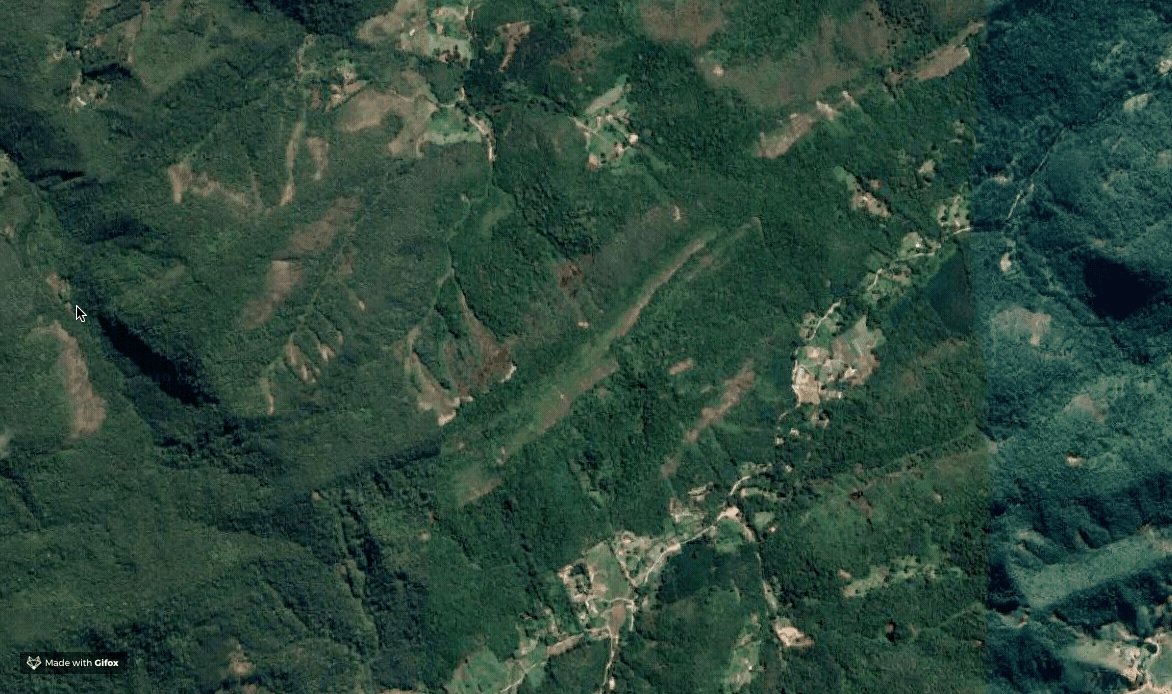This tutorial shows how to generate a binary raster file, broadly used in semantic segmentation problems, with python.### Workflow

``````
import os

import rasterio
from rasterio.plot import reshape_as_image
from rasterio.features import rasterize

import pandas as pd
import geopandas as gpd
from shapely.geometry import mapping, Point, Polygon

import numpy as np
import cv2
import matplotlib.pyplot as plt

``````

#### 1. load .tif image file

Define the raster_path variable with the path to the .tif image. The binary raster will have the same dimensions as this imagem.

``````
raster_path = "/Users/...../imagem.tif"
with rasterio.open(raster_path, "r") as src:
raster_meta = src.meta

``````

#### 2. Load Shapefile or GeoJson

Define the shape_path with the path to the shapefile or the geojson file.

``````
shape_path = "/Users/.../poligono.geojson"

``````

#### 3.Verify the Coordinate Reference System (CRS)

The CRS of the image and the shapefile must be the same. If they aren`t, use QGIS to convert both files to the same CRS.

``````
print("CRS Raster: {}, CRS Vector {}".format(train_df.crs, src.crs))

``````

#### 4. Generate the binary mask

The following code genates the binary mask and plot it.

``````#Generate polygon
def poly_from_utm(polygon, transform):
poly_pts = []

for i in np.array(poly.exterior.coords):

# Convert polygons to the image CRS
poly_pts.append(~transform * tuple(i))

# Generate a polygon object
new_poly = Polygon(poly_pts)
return new_poly

# Generate Binary maks

poly_shp = []
im_size = (src.meta['height'], src.meta['width'])
for num, row in train_df.iterrows():
if row['geometry'].geom_type == 'Polygon':
poly = poly_from_utm(row['geometry'], src.meta['transform'])
poly_shp.append(poly)
else:
for p in row['geometry']:
poly = poly_from_utm(p, src.meta['transform'])
poly_shp.append(poly)

out_shape=im_size)

plt.figure(figsize=(15,15))

``````

#### 5. Save

``````mask = mask.astype("uint16")
save_path = "/Users/.../mascaras/train.tif"
with rasterio.open(save_path, 'w', **bin_mask_meta) as dst:

``````

#### 6. Defining a Function that Generates Binary Masks

The function generate_mask have the following parameters:

raster_path = path to the .tif;

shape_path = path to the shapefile or GeoJson;

output_path = Path to save the binary mask.

file_name = Name of the file.

``````

"""Function that generates a binary mask from a vector file (shp or geojson)

raster_path = path to the .tif;

shape_path = path to the shapefile or GeoJson.

output_path = Path to save the binary mask.

file_name = Name of the file.

"""

with rasterio.open(raster_path, "r") as src:
raster_meta = src.meta

#Verify crs
if train_df.crs != src.crs:
print(" Raster crs : {}, Vector crs : {}.\n Convert vector and raster to the same CRS.".format(src.crs,train_df.crs))

def poly_from_utm(polygon, transform):
poly_pts = []

for i in np.array(poly.exterior.coords):

poly_pts.append(~transform * tuple(i))

new_poly = Polygon(poly_pts)
return new_poly

poly_shp = []
im_size = (src.meta['height'], src.meta['width'])
for num, row in train_df.iterrows():
if row['geometry'].geom_type == 'Polygon':
poly = poly_from_utm(row['geometry'], src.meta['transform'])
poly_shp.append(poly)
else:
for p in row['geometry']:
poly = poly_from_utm(p, src.meta['transform'])
poly_shp.append(poly)

out_shape=im_size)

#Salve